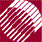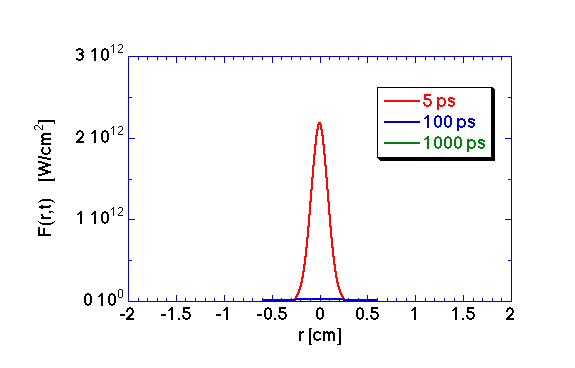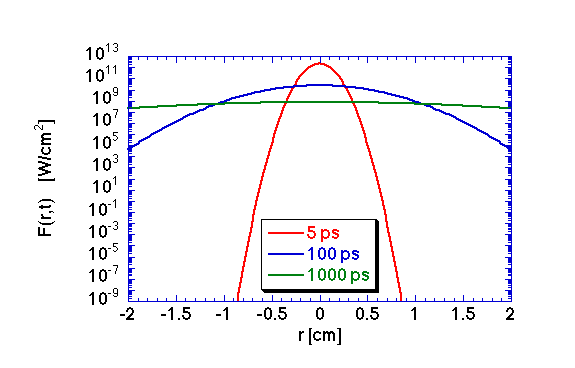ECE532 Biomedical Optics © 1998 Steven L. Jacques, Scott A. Prahl Oregon Graduate Institute

# Time-resolved Diffusion theory

## Examples

The following figures illustrate the time-resolved transport of light from an impulse isotropic point source of energy within a homogeneous unbounded medium with absorption and scattering properties.

The simulation parameters for the medium are µa = 1.0 cm-1, µs(1 - g) = 10.0 cm-1, nt = 1.33. The total impulse energy is Uo = 1 J

The fluence rate F(r,t) is plotted versus r at three timepoints: t = 5, 100, and 1000 ps.

### The time-resolved fluence rate### The same data plotted as a semi-log plotThe C program that generated the data in the above figures is listed here.

to next page | Chapter 5 | Course | Home